# Periodic Motion

A motion that repeats itself at regular intervals of time is called periodic motion.

Note that both the curved parts in Fig (c) are sections of a parabola given by Newton’s equation of motion with different values of u in each case. These are examples of periodic motion.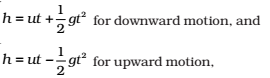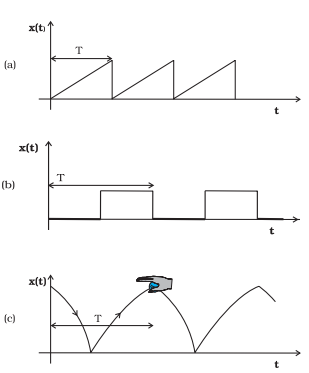Fig. Examples of periodic motion. The period T is shown in each case.

## Oscillations

If the body is given a small displacement from the position, a force comes into play which tries to bring the body back to the equilibrium point, giving rise to oscillations or vibrations.

For example: – A ball placed in a bowl will be in equilibrium at the bottom. If displaced a little from the point, it will perform oscillations in the bowl. Every oscillatory motion is periodic, but every periodic motion need not be oscillatory. Circular motion is a periodic motion, but it is not oscillatory.

There is no significant difference between oscillations and vibrations.

1. When the frequency is small, we call it oscillation (like the oscillation of a branch of a tree)
2. When the frequency is high, we call it vibration (like the vibration of a string of a musical instrument).

## Period and frequency

### Period:

A motion that repeats itself at regular intervals of time is called periodic motion. The smallest interval of time after which the motion is repeated is called its period.

Let us denote the period by the symbol T.

Its SI unit is second.

The period of vibrations of a quartz crystal is expressed in units of microseconds (10-6 s) abbreviated as μs.

### Frequency:

The reciprocal of T gives the number of repetitions that occur per unit time. This quantity is called the frequency of the periodic motion. It is represented by the symbol ν . The relation between v and T isThe unit of ν is thus s-1. It is called hertz (abbreviated as Hz). Thus,

1 hertz = 1Hz = 1 oscillation per second = 1s-1

### Displacement

An oscillating simple pendulum, the angle from the vertical as a function of time may be regarded as a displacement variable [see Fig.(b)]. in the context of position only.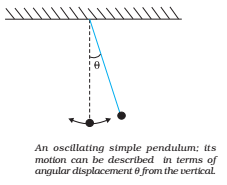There can be many other kinds of displacement variables. The voltage across a capacitor, changing with time in an a.c. circuit is also a displacement variable.

The displacement can be represented by a mathematical function of time. In case of periodic motion, this function is periodic in time.

• One of the simplest periodic functions is given by• If the argument of this function, ωt, is increased by an integral multiple of 2π radians, the value of the function remains the same.
• The function f (t) is the periodic and its period, T, is given by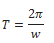• Thus, the function f(t) is periodic with period T.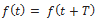• The same result is obviously correct if we consider a sine function,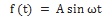• Further, a linear combination of sine and cosine functions like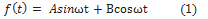is also a periodic function with the same period T. Taking,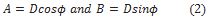Eq.(1) can be written as,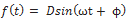• Here D and ϕ are constant given by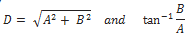Any periodic function can be expressed as a superposition of sine and cosine functions of different time periods with suitable coefficients.